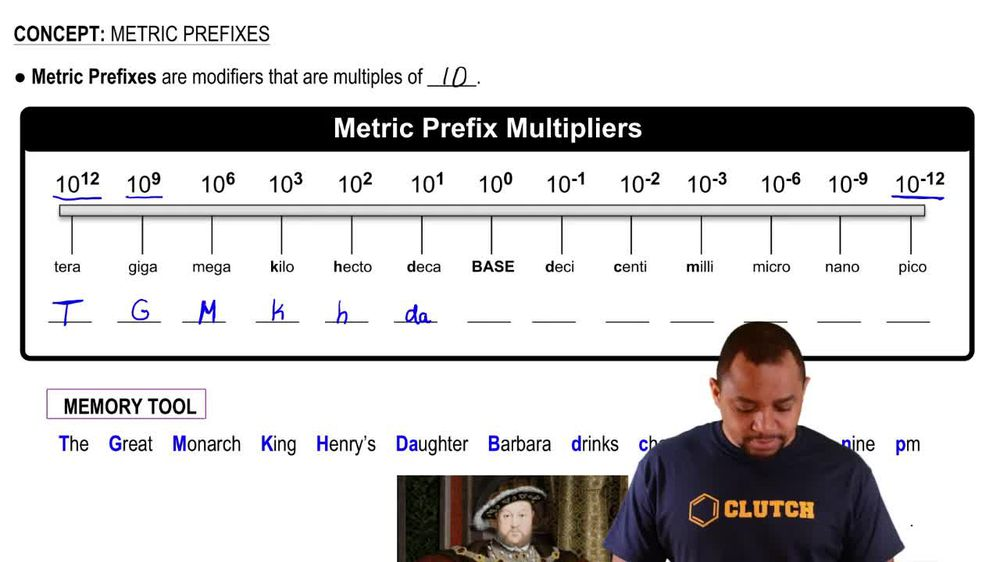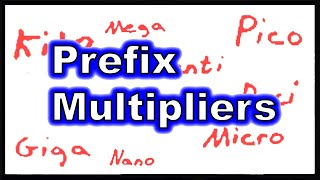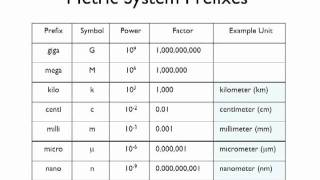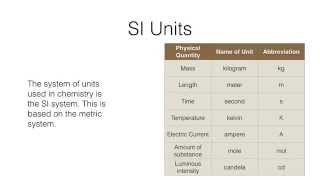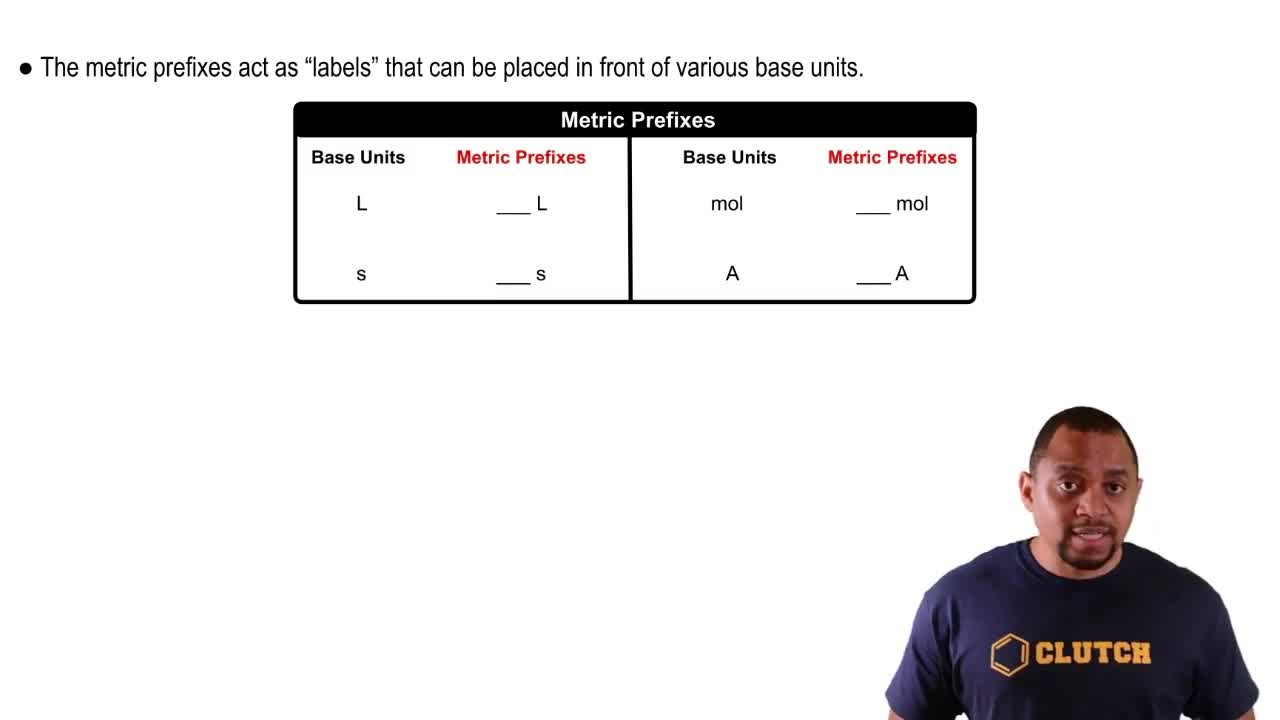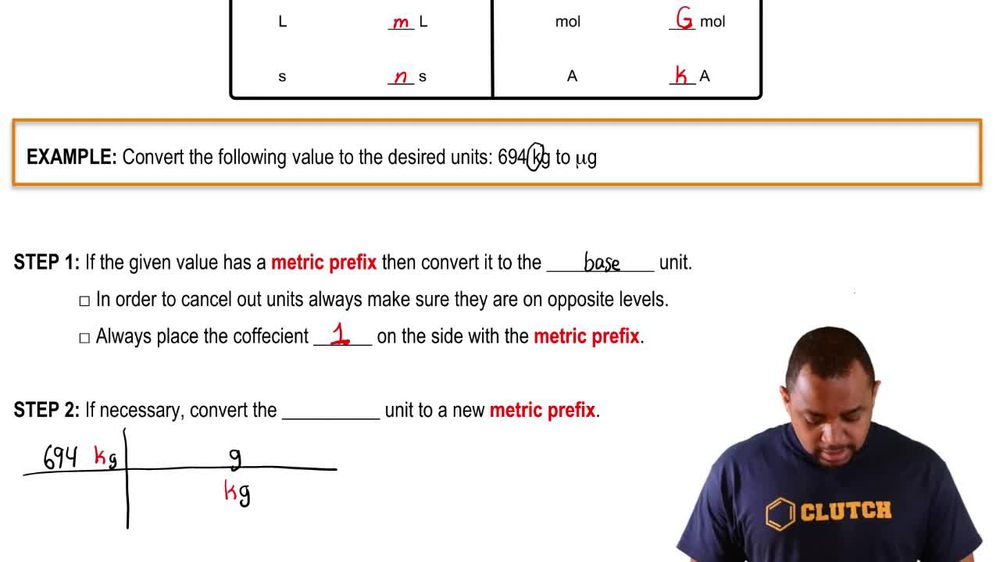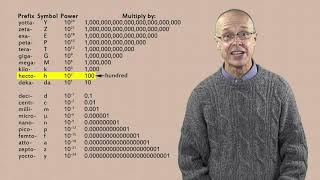Start typing, then use the up and down arrows to select an option from the list.
1. 1. Intro to General Chemistry2. Metric Prefixes
Problem

# Which quantity represents the largest mass? (LO 1.4) (a) 2.5 * 107 mg (b) 2.5 * 102 mg (c) 2.5 * 108 ng (d)2.5*10-3kg

Relevant Solution2m
Play a video:
Hello everyone today. We have the following problem identify the amount that corresponds to the smallest mass. So we're gonna have to go through each one of these since they are in different units, we're going to assess them and convert each of them two g. So we're gonna convert our micro grams two g. So we have 6.4 times 10 to the seventh micrograms. And to convert that to grams we're gonna use a conversion factor. That one microgram is equal to 10 to the negative six g. When the units cancel out we get 64 g and this is for a For B. We have 6.4 times 10 to the 5th micrograms or milligrams. Excuse me. And so we're going to use a conversion factor that there's 10 point negative three g in one mg. Our unit's canceled out. And we're left with 640 g. So we know that b will not be our smallest mass. Moving on to see we have 6.4 times 10 to the negative fourth kilograms. To convert kilograms to regular grams we're gonna use the conversion factor that one kg is equal to 10 to the third grams. And when our units cancel we're left with 0.64 g. Of course A is bigger than option C. So they cannot be our answer. And lastly we have 6.4 times to the ninth nanograms. And to convert nanograms two g. We say that there is for every 10 it's the negative nine g. We have one nanogram and where our units cancel. We're left with 6.4 g. This is not the smallest unit. The small student is our 0.64 or c 6.4 times 10 to the negative four kg. And with that we've answered our question. I hope this helped until next time.